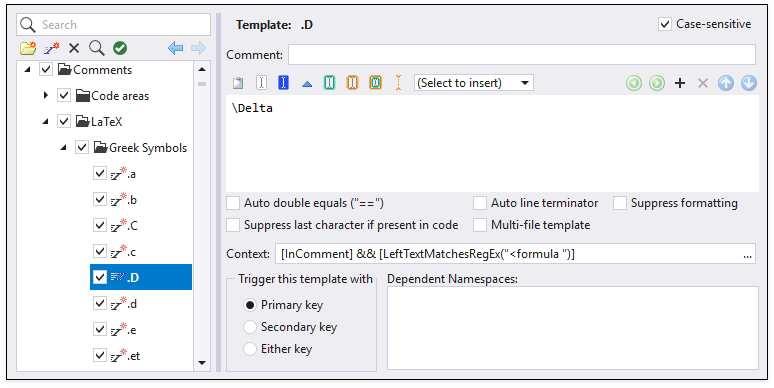# Templates for LaTeX Formula Creation

This topic describes templates you can use to facilitate LaTeX formula creation in Rich Comments.

The LaTeX Formulas Support (beta) feature ships disabled by default. You can enable LaTeX formula support on the Rich Comments options page.

Important

Visual Studio IntelliSense has priority over CodeRush templates. For information on how to prioritize a CodeRush template over Visual Studio IntelliSense, refer to the following topic section: Expand a Template Instead of Visual Studio IntelliSense.

## LaTeX Formula Creation

The following templates create formatted formulas inside code comments:

Template Description Expansion Example
/f LaTeX formula in C#. // <formula >
‘f LaTeX formula in VB. ‘ <formula >
!f LaTeX formula in XAML. <!– <formula > –>
\. Centered dot \cdot\.. Three centered dots \cdots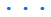\8 Infinity \infty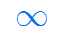\b Braces {}
\bca Big Cap \bigcap_{lower}^{upper}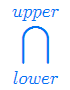\bcu Big Cup \bigcup_{lower}^{upper}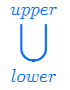\cp Co-product \coprod_{lower}^{upper}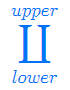\f Fraction \frac{numerator}{denominator}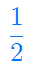\l Limit \lim_{x\to\infty}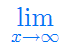\lr Left & Right Parens \left( \right)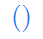\nr nth Root \sqrt[root]{value}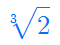\o Circle symbol \circ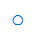\oi Contour integral \oint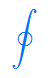\p Product \prod_{lower}^{upper}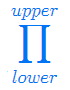\s Sum \sum_{lower}^{upper}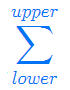\sq Square Root \sqrt{value}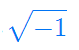\v Vector \vec{numerator}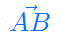## Example

The screencast below demonstrates how to use “/f”, “\f” and “\sq” templates to create a quadratic formula in rich comments.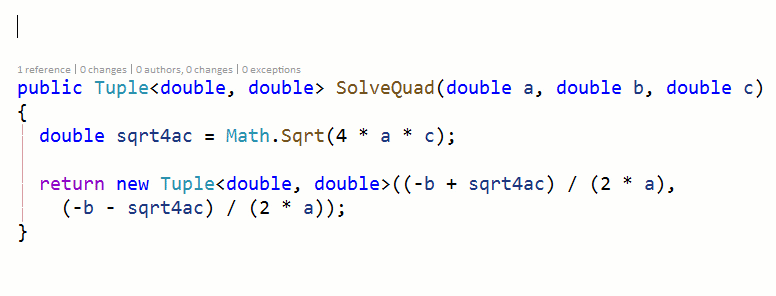## Greek Symbols in LaTeX Formula

CodeRush supports Greek letters in LaTeX formulas.

### How to Use

To use a Greek letter in a formula, enter a “.” followed by the letter (or letter abbreviation).

For example, to reference the Greek alpha, use “.a”.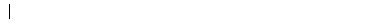Use an uppercase letter after the dot to get an uppercase Greek letter.

For example, use “.D” to get an uppercase Delta symbol.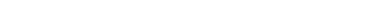### Template Configuration

You can find a complete list of supported Greek symbols on the CodeRush Templates options page.